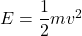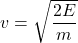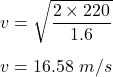## A 1.6 Kg bird that is flying through the air has 220 J of kinetic energy. How fast is the bird flying? 20.5 m/s 19.4 m/s

Question

A 1.6 Kg bird that is flying through the air has 220 J of kinetic energy. How fast is the bird flying?

20.5 m/s
19.4 m/s
18.2 m/s
16.6 m/s
cuh

in progress 0
2 months 2021-07-25T05:31:38+00:00 1 Answers 3 views 0

v = 16.58 m/s

Explanation:

Given that,

Mass of a bird, m = 1.6 kg

Kinetic energy of the bird, K = 220 J

We need to find the speed of the bird. The formula for the kinetic energy is given by :Where

v is the speed of the bird

So,Put all the values,So, the speed of the bird is equal to 16.58 m/s.